Next: Quantum Electrodynamics Involving a Up: Laser Previous: Laser Geometry

### Linear Compton Scattering

When the parameter NPH=0 is specified in LASERQED command, the formulas of linear Compton scattering are used.

Let us define the following variables in the rest frame of the initial electron: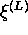,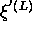Photon Stokes parameters before and after collision as defined in(page 361).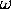,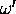Initial (laser) and final energies of the photon.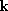,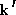Initial (laser) and final momenta of the photon.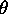,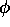Polar and azimuthal scattering angle of the photon.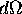Solid angle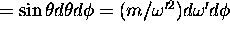.

The range ofis given by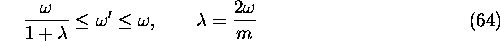The Compton relation is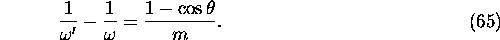The crosssection is given by eq(87.22) in .: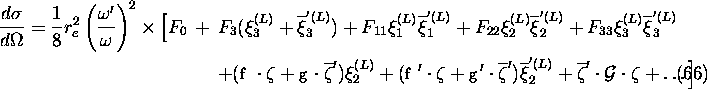See Sec.5.2.2 for the meaning of the bars on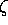and. The omitted terms are products of three and four among,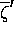,, and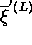. (Actually, we need the terms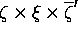and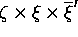but they are not found in literature.) The functions introduced in the above expression are: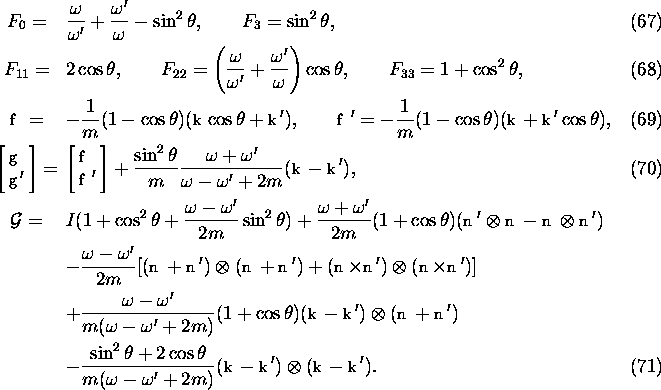These formulas are used in their exact forms in CAIN.

Summation over the final polarization and the azimuthal anglegives the differential crosssection with respect to the final photon energy. Introducing the variables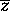inplace ofby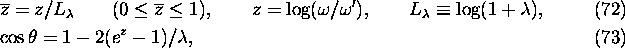we write the differential crossection as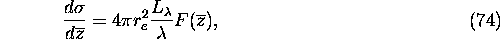where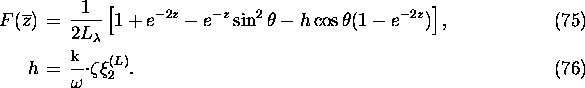Note that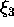does not appear here because it is based on the scattering plane and, therefore, disappears after integration over the azimuthal angle. The function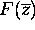satisfies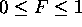for anyand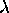and is O(1) except when h is close to +1 andis extremely large. The Functionis plotted in Fig.5.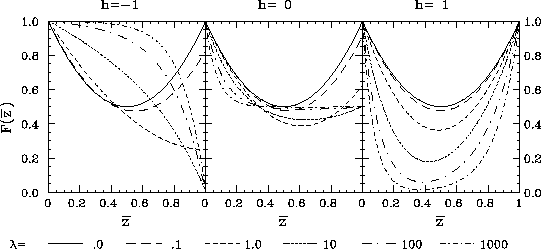Figure 5: Functionfor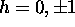for various values ofThe total crosssection for given initial momenta and polarizations is given by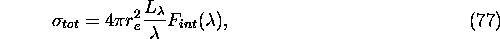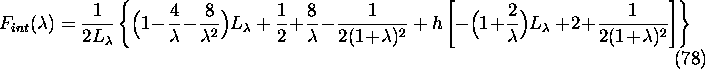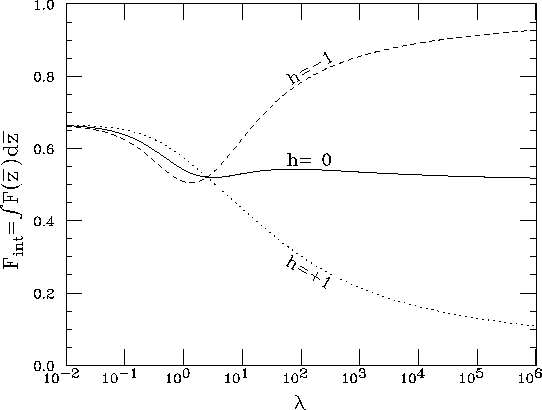Figure 6: Function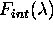foras a function of.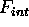is less than unity and is O(1) unless h is close to +1 andis extremely large.

Let us briefly describe the algorithm of event generation.

1. Compute the total event rate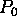in the given time interval using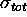without the factor. Since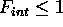, this is an over estimation of the rate. Ifis too large, divide the time interval by an integer N and repeat the following procedure N times.
2. Generate a random number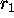uniform in (0,1). Reject if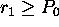.
3. Computeand multiply it to. Reject if still. Otherwise accept. Note that the Lorentz transformation ofis not needed for the computation of h because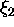is Lorentz invariant. Also note that inputis defined already in the rest frame of electron. Only the Lorentz transformation ofis needed.
4. Generate two random numbersand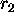in (0,1). Repeat this step until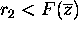is satisfied. Once or twice repetition is normally enough unless h is close to +1 andis very large.
5. Computefrom. Generate the azimuthal angle, compute the final polarization if needed, and go back to the laboratory frame. In this step many Lorentz transformations are needed.Next: Quantum Electrodynamics Involving a Up: Laser Previous: Laser Geometry

Toshiaki Tauchi
Thu Dec 3 17:27:26 JST 1998